# 1. 层次聚类

层次聚类（hierarchical clustering）试图在不同层次对数据集进行划分，从而形成树形的聚类结构。数据集的划分可采用“自底向上”的聚合策略，也可采用“自顶向下”的分拆策略。

## 1.1 凝聚聚类

凝聚聚类（Agglomerative Clustering）是一种采用自底向上聚类策略的层次聚类算法。它先将数据集中的每个样本看作一个初始聚类簇，然后在算法运行的每一步中找出距离最近的两个聚类簇进行合并。该过程不断重复，直到达到预设的聚类簇个数。这里的关键是如何计算聚类簇之间的距离。实际上，每个簇是一个样本集合，因此，只需要采用关于集合的某种距离即可。例如，给定聚类簇 $C_i$$C_j$，可通过下面的式子来计算距离：

$最小距离：d_{\min }\left(C_{i}, C_{j}\right)=\min _{\boldsymbol{x} \in C_{i}, \boldsymbol{z} \in C_{j}} \operatorname{dist}(\boldsymbol{x}, \boldsymbol{z}) \tag{1}$

$最大距离：d_{\max }\left(C_{i}, C_{j}\right)=\max _{\boldsymbol{x} \in C_{i}, \boldsymbol{z} \in C_{j}} \operatorname{dist}(\boldsymbol{x}, \boldsymbol{z}) \tag{2}$

$平均距离：d_{\operatorname{avg}}\left(C_{i}, C_{j}\right)=\frac{1}{\left|C_{i}\right|\left|C_{j}\right|} \sum_{\boldsymbol{x} \in C_{i}} \sum_{\boldsymbol{z} \in C_{j}} \operatorname{dist}(\boldsymbol{x}, \boldsymbol{z}) \tag{3}$

$离差平方和：ESS=\sum_{i=1}^n x_i^2-\frac{1}{n}\big(\sum_{i=1}^n x_i\big)^2 \tag{4}$

$\Delta(C_i,C_j)=ESS(C_i \cup C_j)-ESS(C_i)-ESS(C_j) \tag{5}$

• 在第 1-9 行，算法先对仅包含一个样本的初始聚类簇和相应的距离矩阵进行初始化；

• 在第 11-23 行，凝聚算法不断合并距离最近的聚类簇，并对合并得到的聚类簇的距离矩阵进行更新；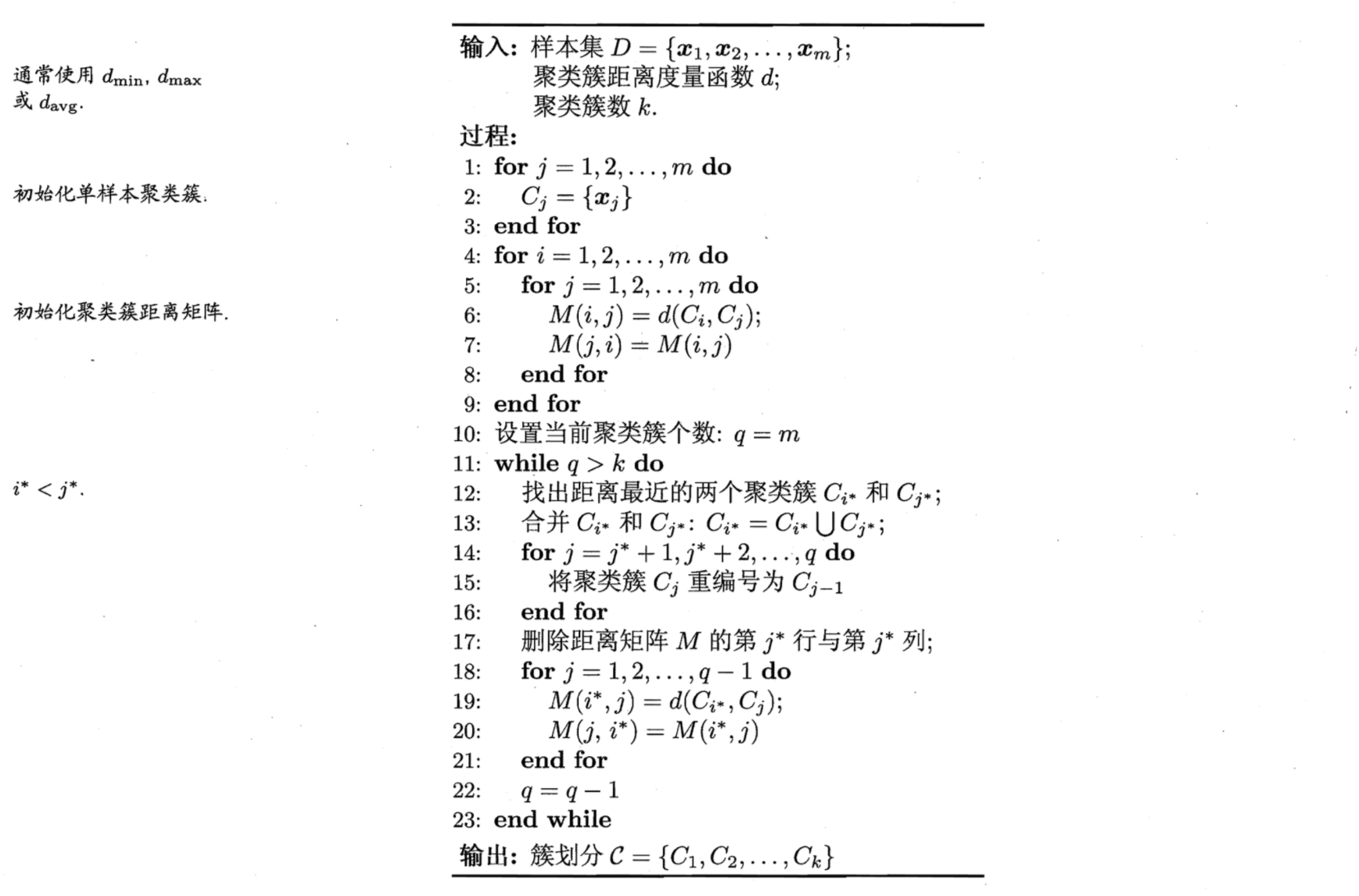## 1.2 层次图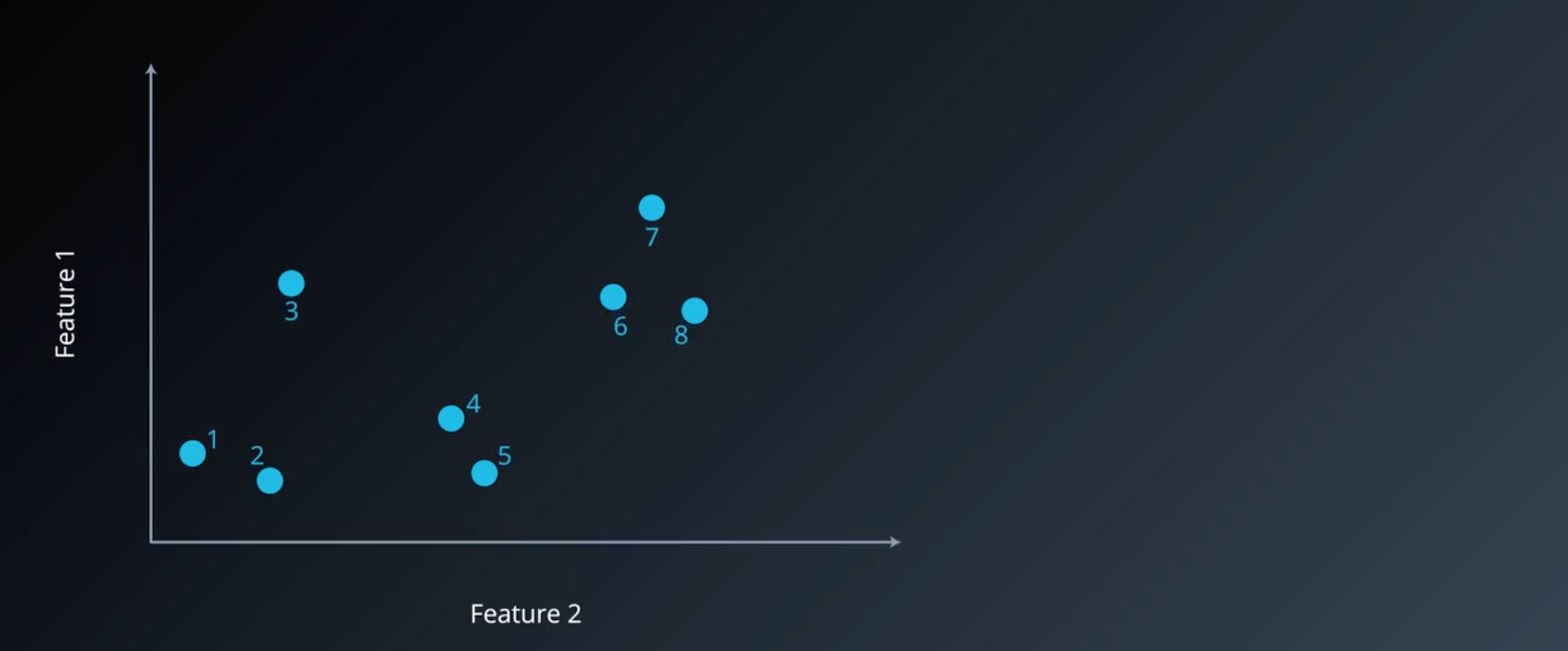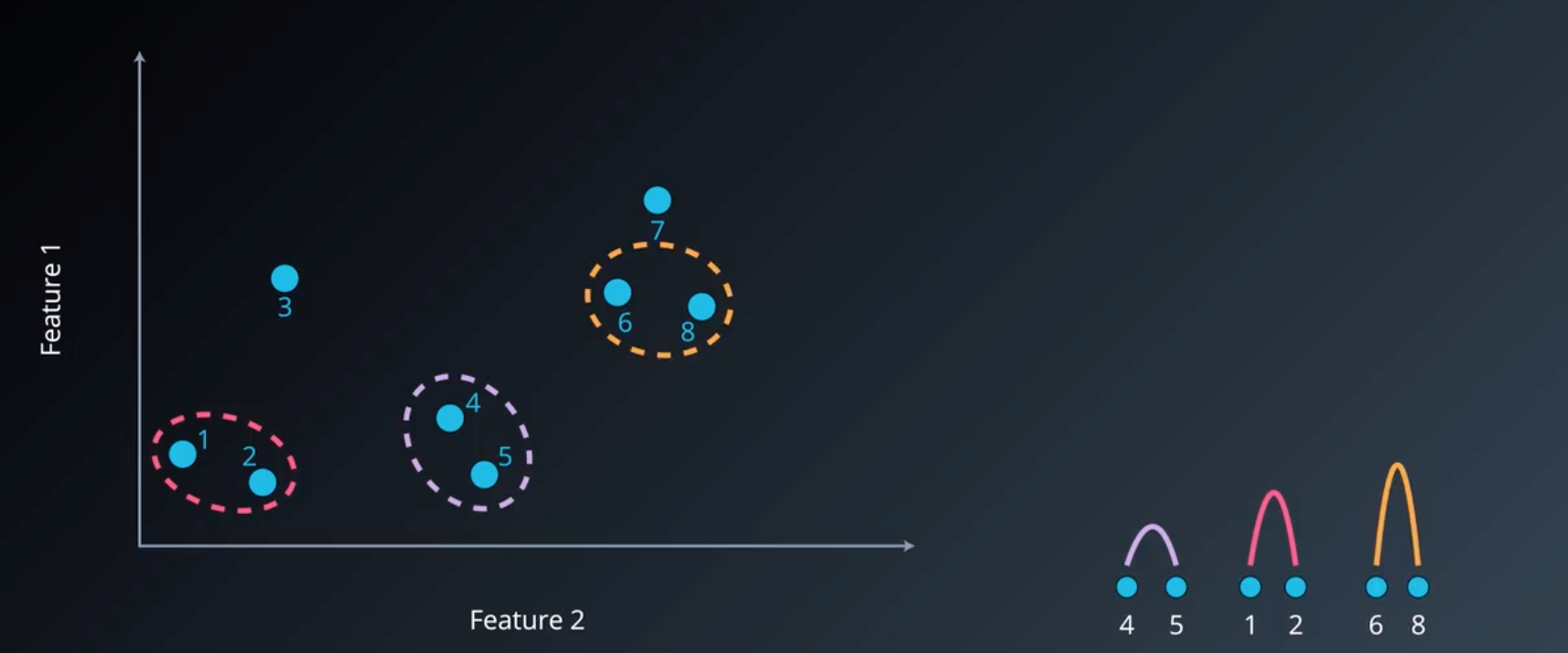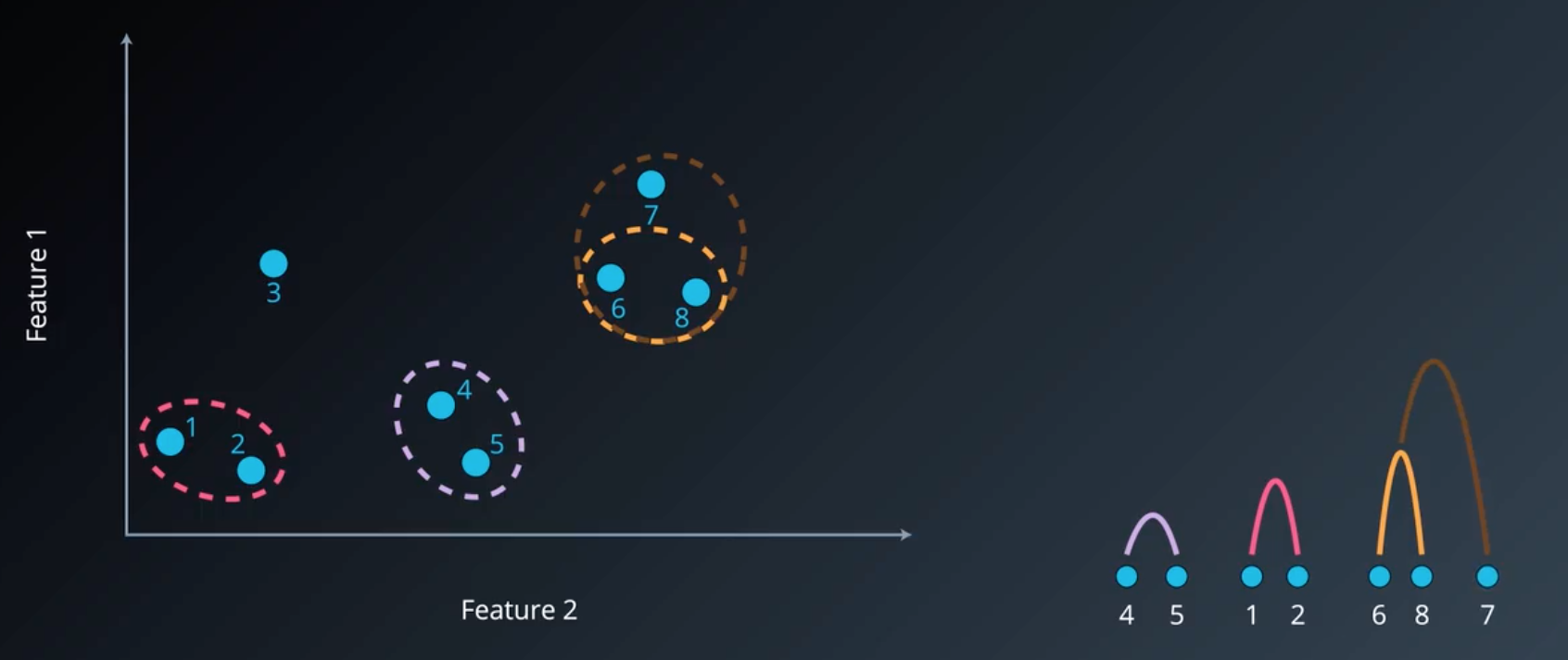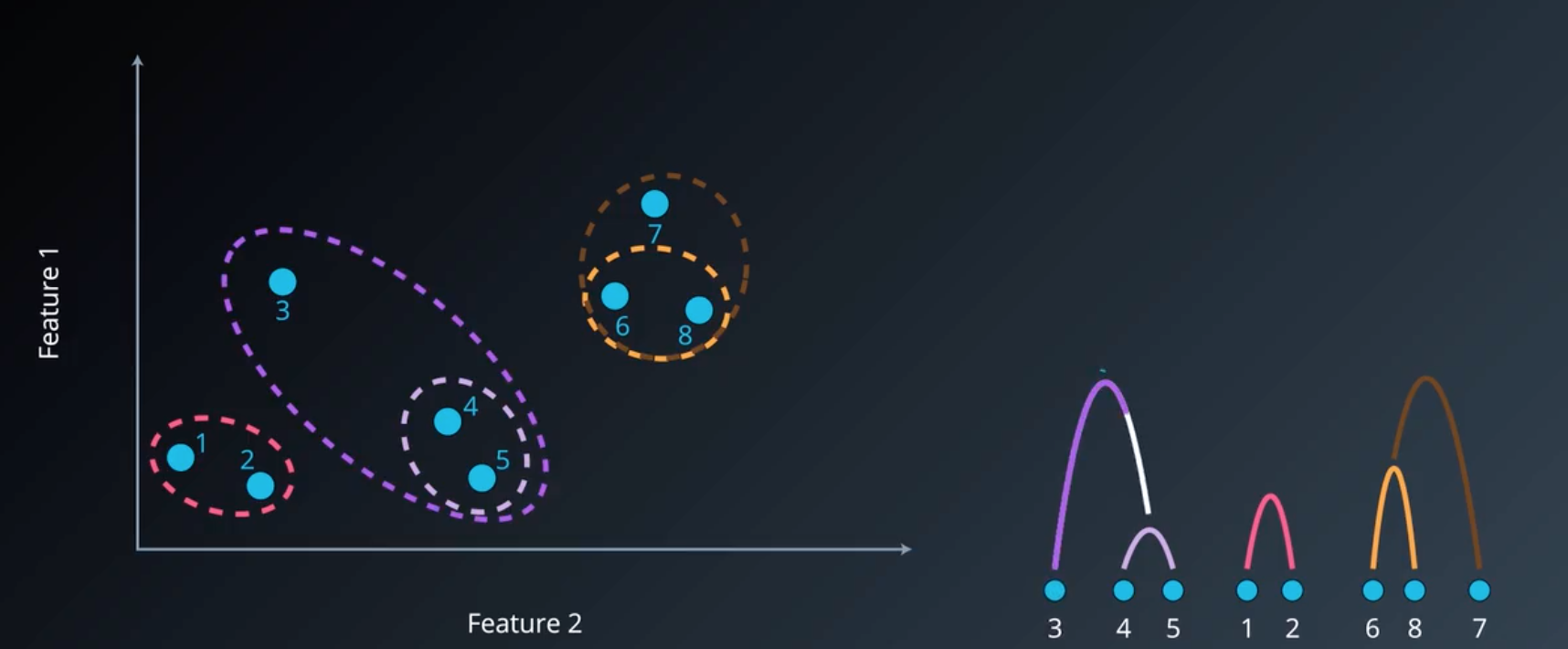## 1.3 不同凝聚算法比较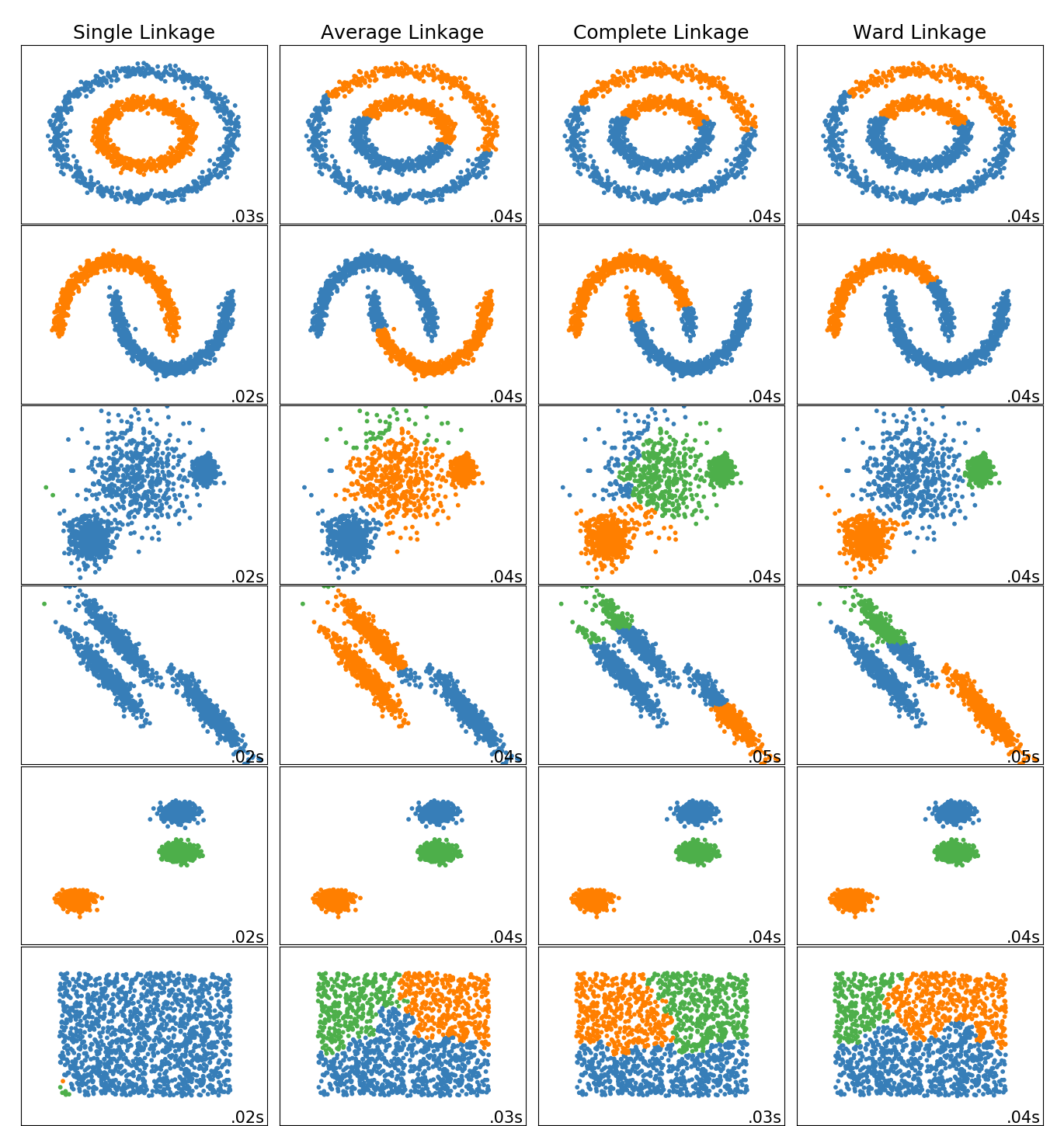# 2. Sklearn 实现

sklearn.cluster.AgglomerativeClustering(n_clusters=2, affinity=‘euclidean’, memory=None, connectivity=None, compute_full_tree=‘auto’, linkage=‘ward’, distance_threshold=None)

n_clusters：聚类簇数

affinity： string or callable, default: “euclidean” 【距离计算参数】

Metric used to compute the linkage. Can be “euclidean”, “l1”, “l2”, “manhattan”, “cosine”, or “precomputed”. If linkage is “ward”, only “euclidean” is accepted. If “precomputed”, a distance matrix (instead of a similarity matrix) is needed as input for the fit method.

linkage： {“ward”, “complete”, “average”, “single”}, optional (default=”ward”)

Which linkage criterion to use. The linkage criterion determines which distance to use between sets of observation. The algorithm will merge the pairs of cluster that minimize this criterion.

• ward minimizes the variance of the clusters being merged.

• average uses the average of the distances of each observation of the two sets.

• complete or maximum linkage uses the maximum distances between all observations of the two sets.

• single uses the minimum of the distances between all observations of the two sets.

## 2.1 层次图可视化

from scipy.cluster.hierarchy import dendrogram, ward, single
import matplotlib.pyplot as plt

plt.show


<function matplotlib.pyplot.show(*args, **kw)>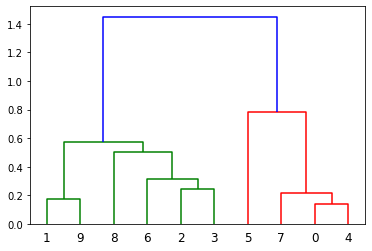# 参考文献

 周志华. 机器学习[M]. 北京: 清华大学出版社, 2016: 214.

 Ward J H , Jr. Hierarchical Grouping to Optimize an Objective Function[J]. Journal of the American Statistical Association, 1963, 58(301):236-244.©️2019 CSDN 皮肤主题: 大白 设计师: CSDN官方博客